# Assignments

The purpose of this series of assignments is to play with trigonometric functions. We use some formulas we already used in the topic Trigonometry Unit circle and simple formulas. There are also other heavily used formulas: the so-called addition and subtraction formulas. Especially in this case we know: practice makes perfect.

1. Show: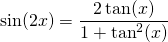Solution

2. Show: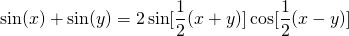Solution

3. Solve for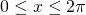: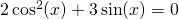Solution

4. Use the Simpson/Mollweide formulas for the following formula: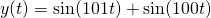Solution

5. Show the following relation using some trigonometric formulas: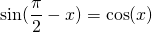Solution

6. Show: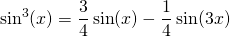Solution

7. Solve for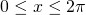: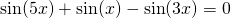Solution

8. A point moves in the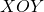-plane. It moves in the-direction according to the function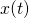and in the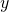-direction according to the function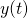. When we have: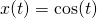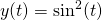calculate the equation of the curve along which the point is moving.

Solution

9. Show:Solution

10. Show: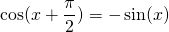Solution

0